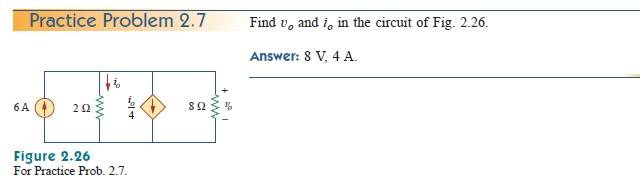# Simple circuit.

• Engineering

## Homework Statement## Homework Equations

I am trying to do some self study here before I take my circuits course. I am stuck on this problem for some reason.. The equation I am coming up with for the node is : 6 - Vo/2 - Io/4 - Vo/8 = 0.. After getting at equation I cant seem to come to the answer. Do I need to do another equation here? Thanks

## The Attempt at a Solution

cepheid
Staff Emeritus
Gold Member
Welcome to PF Would!

## Homework Statement## Homework Equations

I am trying to do some self study here before I take my circuits course. I am stuck on this problem for some reason.. The equation I am coming up with for the node is : 6 - Vo/2 - Io/4 - Vo/8 = 0.. After getting at equation I cant seem to come to the answer. Do I need to do another equation here? Thanks

## The Attempt at a Solution

Ohm's law for the 2 ohm resistor tells you that i0 = v0/(2 Ω). That's your second equation. Now you have everything in terms of only one unknown, and you can therefore solve the system.

Thanks a lot for the help! I must be doing some simple math wrong here.. but im getting : 6 - Vo/2 - Vo/4 - Vo/8 = 0 then multiplying all of that by 8 to get 48- 4Vo - 2Vo - Vo = 0 then ultimately getting Vo = 6.85V but that is wrong... What am I doing wrong there? Should I be assuming that the current in across the 8 ohm resistor is moving upward and therefore making the equation 48 - 4Vo - 2Vo + Vo = 0? It works out that way but if it is like that could you tell me why? Since it is not marked i assumed it was a voltage drop across the 8 ohm resistor..

cepheid
Staff Emeritus